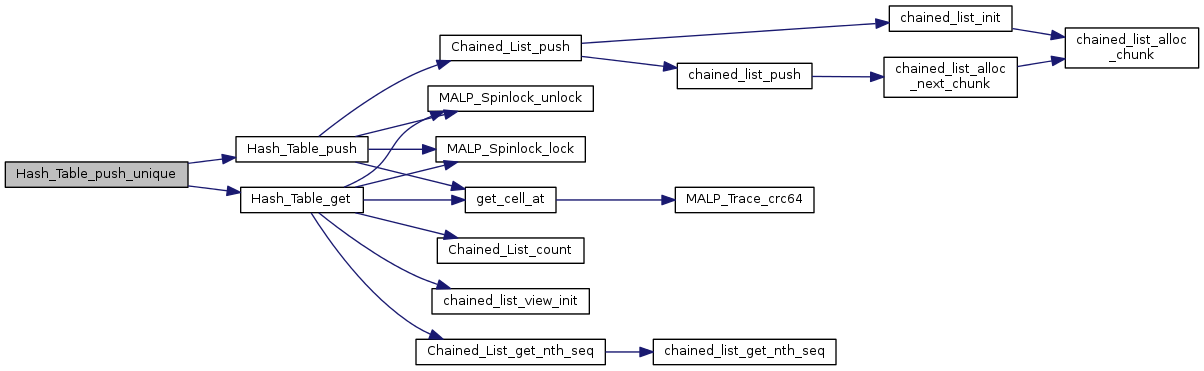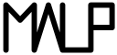Multi-ApplicationOnlineProfiling  2.1
Accessors

Accessors functions (push, get, etc.) More...

## Functions

void * Hash_Table_get (struct Hash_Table *ht, uint64_t key, void *extra_arg, int(*test_func)(void *, uint64_t, void *))
retrieves an element from the hashtable More...

void * Hash_Table_push (struct Hash_Table *ht, void *payload, uint64_t key)
adds an element to a hashtable More...

void * Hash_Table_get_an_entry (struct Hash_Table *ht)
Retrieves the first found element of the hash table. More...

void * Hash_Table_push_unique (struct Hash_Table *ht, void *payload, uint64_t key, void *extra_arg, int(*test_func)(void *, uint64_t, void *))
Adds an element into the hashtable. Replaces already existing element at key. More...

uint64_t Hash_Table_count_by_walk (struct Hash_Table *ht)
counts the elements in the hashtable by walking through all the elements More...

## Detailed Description

Accessors functions (push, get, etc.)

## Function Documentation

 uint64_t Hash_Table_count_by_walk ( struct Hash_Table * ht )

counts the elements in the hashtable by walking through all the elements

Parameters
 ht where to count elements
Returns
the number of elements

Definition at line 425 of file Hash_Table.c.

Here is the call graph for this function: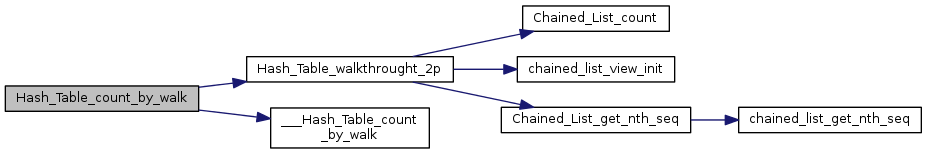void* Hash_Table_get ( struct Hash_Table * ht, uint64_t key, void * extra_arg, int(*)(void *, uint64_t, void *) test_func )

retrieves an element from the hashtable

Parameters
 ht where to retrieve the element key the key of the wanted element extra_arg extra argument to pass to test_func test_func key comparison function. Takes a pointer to stored data, the key and an extra arg. returns 1 if test success
Returns
the found element

Definition at line 128 of file Hash_Table.c.

Here is the call graph for this function: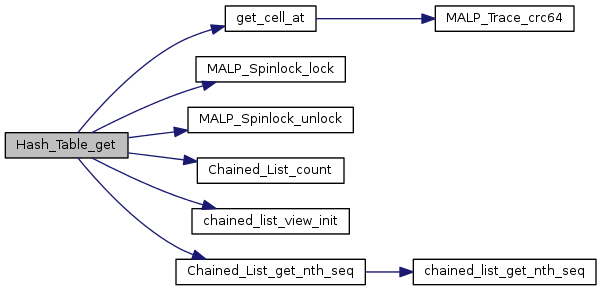Here is the caller graph for this function: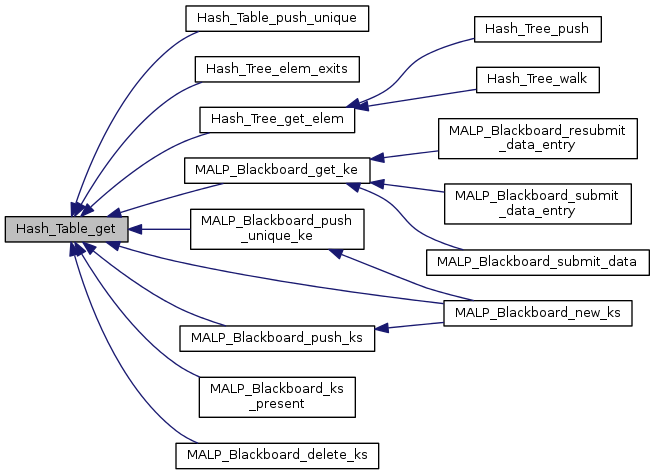void* Hash_Table_get_an_entry ( struct Hash_Table * ht )

Retrieves the first found element of the hash table.

Parameters
 ht the hash table where to get the element
Returns
the element (NULL if the table is empty)
Warning
Only used in Unit Tests and Red_Ht_Table_get_an_entry() (which is never used...)

Definition at line 329 of file Hash_Table.c.

 void* Hash_Table_push ( struct Hash_Table * ht, void * payload, uint64_t key )

adds an element to a hashtable

Parameters
Returns

Definition at line 198 of file Hash_Table.c.

Here is the call graph for this function: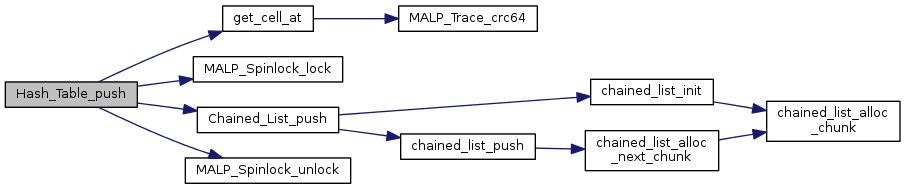Here is the caller graph for this function: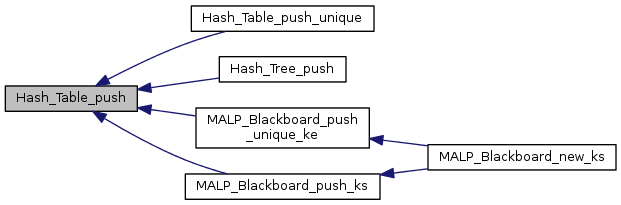void* Hash_Table_push_unique ( struct Hash_Table * ht, void * payload, uint64_t key, void * extra_arg, int(*)(void *, uint64_t, void *) test_func )

Adds an element into the hashtable. Replaces already existing element at key.

Parameters
 ht where to add the element payload the element to add key where to add it extra_arg extra argument to pass to test_func test_func the same test function used with Hash_Table_get()
Returns